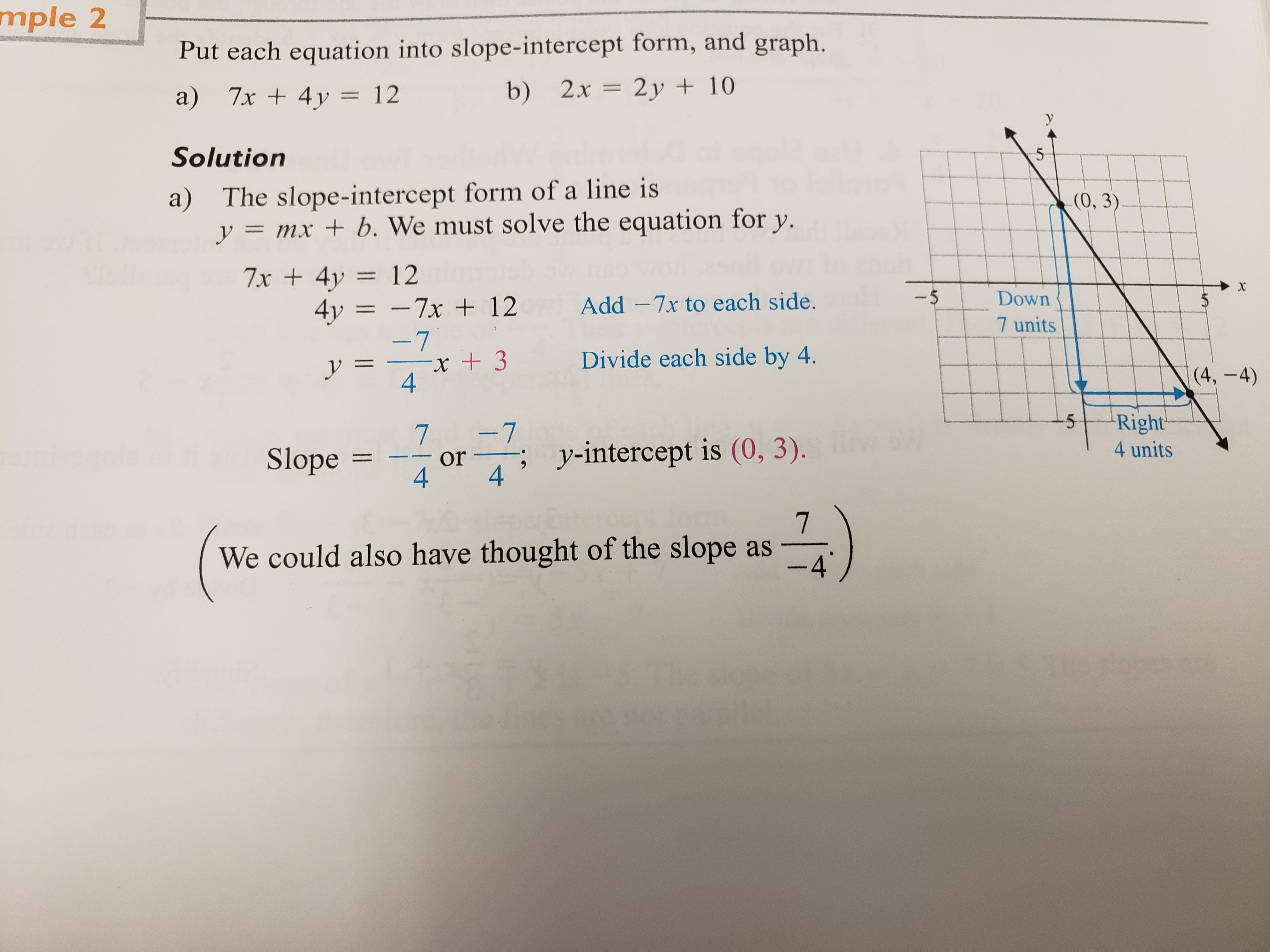# mple 2Put each equation into slope-intercept form, and grapha) 7x 4y 12Solutiona) The slope-intercept form of a line isb) 2x 2y 10(0, 3ymxb. We must solve the equation for y.4y7x 12 Add -7x to each side.Down7 units+3Divide each side by 44Slope 7or vinteropt is 0.3)Right4 units4We could also have thought of the slope as-4

Question

How do I rewrite an equation in slope intercept form and graph the line?

Example they gave was 7x + 4y=12 to be written as y=mx+b

I dont understand how adding -7 to each side ended up being -7/4x

Thank you kindly for helpinghelp_outlineImage Transcriptionclosemple 2 Put each equation into slope-intercept form, and graph a) 7x 4y 12 Solution a) The slope-intercept form of a line is b) 2x 2y 10 (0, 3 ymx b. We must solve the equation for y. 4y7x 12 Add -7x to each side. Down 7 units +3 Divide each side by 4 4 Slope 7or vinteropt is 0.3) Right 4 units 4 We could also have thought of the slope as -4 fullscreen
check_circle

star
star
star
star
star
1 Rating
Step 1

a)

Given:

The equation is 7x + 4y = 12.

Known fact:

Slope-intercept form of an equation of a line:

An equation of a line with slope m and y-intercept b is .”

The slope m of the non-vertical line can be expressed as follows.

That is, the slope of the line represents the rate of change of y with respect to the changes in x.

Step 2

Calculation:

The slope and the y-intercept is computed as follows.

Rewrite the given equation as

Step 3

Since 3 is the y-intercept, one of the point on the line will be (0,3) .

Compu...

### Want to see the full answer?

See Solution

#### Want to see this answer and more?

Solutions are written by subject experts who are available 24/7. Questions are typically answered within 1 hour.*

See Solution
*Response times may vary by subject and question.
Tagged in

### Other